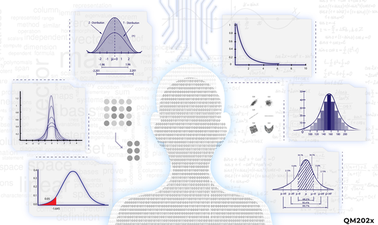Ir al contenido principal

# Foundations of Data Science

Learn the fundamental concepts in probability, statistics, optimization and linear algebra which form the foundations for data science.4–5 horas por semana
Al ritmo del instructor
Con un cronograma específico
Gratis
Verificación opcional disponible

# Sobre este curso

Omitir Sobre este curso

Data Science along with artificial intelligence (AI) and its various components such as statistical learning (SL), machine learning (ML) and deep learning algorithms (DL) are recognized as main drivers of organizational value creation. According to Dr Jim Gray, Data Science is the fourth paradigm which drives innovative solutions to organizational problems.

In this course we will start with basic concepts in probability such as joint and conditional probabilities. We will discuss the implementation of these concepts in ML algorithms for Market Basket Analysis and Recommender Systems. After covering basic probability concepts, we move on to random variables, discrete and continuous probability distributions, sampling, estimation and central limit theorem.

An important step in ML model building is feature selection to avoid overfitting and underfitting. ML models such as regression and logistic regression use hypothesis testing to select features. We will discuss various hypothesis tests and how they are used in feature selection.

Every ML model has an optimization stage, either to fine-tune the feature weights, or to find an optimal set of features. We will discuss important optimization techniques, and algorithms such as Gradient Descent, that play an important role in AI and ML model development.

Data must be represented in a matrix for AI and ML model development. Matrix operations such as matrix inverse and multiplication are elementary steps in model development. These fundamental concepts in linear algebra will be discussed.

This course is suitable for students/practitioners interested in improving their knowledge in the fundamental concepts of Data Science. The course will also prepare the learner for a career in the field of Data Analytics.

### De un vistazo

• Idioma: English
• Transcripción de video: English

# Lo que aprenderás

Omitir Lo que aprenderás
• Describe the role of probability theory, optimization and linear algebra in the field of Artificial Intelligence.
• Define probability distributions such as binomial and normal and its applications in ML model development.
• Conduct hypothesis tests such as Z test and t-test and how it is used in ML Model development.
• Explain optimization and linear algebra concepts and their applications in ML and AI.
• Conduct hypothesis testing, optimization and linear algebra using Excel.

# Acerca de los instructores

## ¿Te interesa este curso para tu negocio o equipo?

Capacita a tus empleados en los temas más solicitados con edX para Negocios.